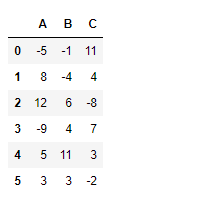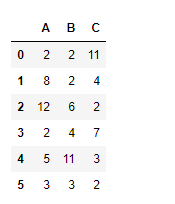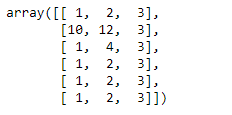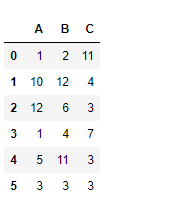Related Articles

# Python | Pandas dataframe.clip_lower()

• Last Updated : 20 Nov, 2018

Python is a great language for doing data analysis, primarily because of the fantastic ecosystem of data-centric python packages. Pandas is one of those packages and makes importing and analyzing data much easier.

Pandas` dataframe.clip_lower()` is used to trim values at specified input threshold. We use this function to trim all the values below the threshold of the input value.

Syntax: DataFrame.clip_lower(threshold, axis=None, inplace=False)

Parameters:
threshold : numeric or array-like
`float `: every value is compared to threshold.
`array-like` : The shape of threshold should match the object it’s compared to. When self is a Series, threshold should be the length. When self is a DataFrame, threshold should 2-D and the same shape as self for axis=None, or 1-D and the same length as the axis being compared.
axis : Align self with threshold along the given axis.
inplace : Whether to perform the operation in place on the data.

Returns: clipped : same type as input

Example #1: Use `clip_lower()` function to trim values of a data frame below a given threshold value.

 `# importing pandas as pd``import` `pandas as pd`` ` `# Creating a dataframe using dictionary``df ``=` `pd.DataFrame({``"A"``:[``-``5``, ``8``, ``12``, ``-``9``, ``5``, ``3``],``                   ``"B"``:[``-``1``, ``-``4``, ``6``, ``4``, ``11``, ``3``], ``                   ``"C"``:[``11``, ``4``, ``-``8``, ``7``, ``3``, ``-``2``]})`` ` `# Printing the data frame for visualization``df`Now trim all the values below 2 to 2.

 `# Clip all values below 2``df.clip_lower(``2``)`

Output :Example #2: Use `clip_lower()` function to clips values in a dataframe with specific value for each cell of the dataframe.

For this purpose, we can use a numpy array, but the shape of array must be same as that of the dataframe.

 `# importing pandas as pd``import` `pandas as pd`` ` `# Creating a dataframe using dictionary`` ` `df ``=` `pd.DataFrame({``"A"``:[``-``5``, ``8``, ``12``, ``-``9``, ``5``, ``3``], ``                   ``"B"``:[``-``1``, ``-``4``, ``6``, ``4``, ``11``, ``3``],``                   ``"C"``:[``11``, ``4``, ``-``8``, ``7``, ``3``, ``-``2``]})`` ` `# lower limit for each individual column element.``limit ``=` `np.array([[``1``, ``2``, ``3``], [``10``, ``12``, ``3``], [``1``, ``4``, ``3``],``                  ``[``1``, ``2``, ``3``], [``1``, ``2``, ``3``], [``1``, ``2``, ``3``]])`` ` `# Print lower_limit``limit`Now apply these limits on the dataframe

 `# applying different limit value ``# for each cell in the dataframe``df.clip_lower(limit)`

Output :Each cell value has been trimmed based on the corresponding lower limit applied.

Attention geek! Strengthen your foundations with the Python Programming Foundation Course and learn the basics.

To begin with, your interview preparations Enhance your Data Structures concepts with the Python DS Course. And to begin with your Machine Learning Journey, join the Machine Learning – Basic Level Course

My Personal Notes arrow_drop_up